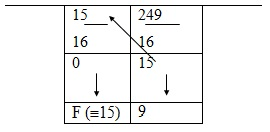0

Hexadecimal number system uses 16 as its base or radix. The basic elements of this system consist of digits from 0 to 9 and alphabets A, B, C, D, E, and F. Letter A to F are assigned with values of 10 to 15. Thus A ≡ 10, B ≡ 11, C ≡ 12, D ≡ 13, E ≡ 14, and F ≡ 15. In terms of binary equivalents, A ≡ 1010, B ≡ 1011, C ≡ 1100, D ≡1101, E ≡ 1110, and F ≡ 1111. Hexadecimal system is used in microprocessors and microcontrollers to write their operating codes (op-codes). Since there are sixteen basic numbers to play with, instructions using hex are more compact than those the using decimal or binary number systems.

Decimal Number to Hexadecimal Number Conversion:
Example: Convert (249)10 to hex.
Solution: (See Fig.1.13)Fig.1.13   Conversion of (249)10 to HEX
From Fig.1.13, we have
(249)10 º (F9)16
The 9’s-complement pairs in this family are (0,9), (1,8), (2,7), (3,6) and (4,5), respectively, since the sum of the numbers in each bracket amounts to 9.  We also get the 10’s complements by adding +1 to the 9’s complements. For example, 4 is the 10’s complement of 6.